# AP Calculus AB Practice Test 12

### Test Information10 questions20 minutes

Calculator Disallowed

1.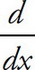tan2(4x) =

2. What is the equation of the line tangent to the graph of y = sin2x at x =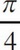?

3. If the function f(x) =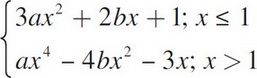is differentiable for all real values of x, then b =

4. The graph of y = x4 + 8x3 - 72x2 + 4 is concave down for

5.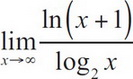6.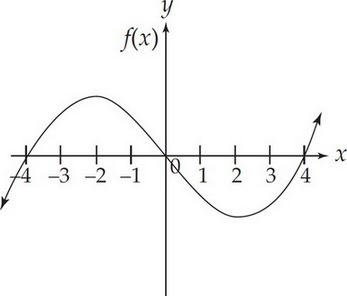The graph of f(x) is shown in the figure above. Which of the following could be the graph of f′(x) ?

7. If f(x) = ln(cos(3x)), then f′(x) =

8. If f(x) =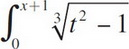, then f′(-4) =

9. A particle moves along the x-axis so that its position at time t, in seconds, is given by x(t) = t2 - 7t + 6. For what value(s) of t is the velocity of the particle zero?

10.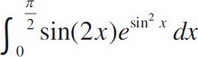=